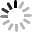Up to 5 files, each 10M size is supported. OK
SICHUAN VSTAR OPTICAL TECHNOLOGY CO.,LTD 86-028-64192839-802 sales@vstarsc.com

### News

Get a QuoteHome - News - What is an ideal optical system?
Services

# What is an ideal optical system?

June 4, 2021

What is an ideal optical system?

In geometric optics, the so-called ideal optical system is an optical system that can form perfect image and ideal image of each point in a large enough space with a wide enough beam.Ideal optical system transfer object space with otimista beam to like one beam of the space, this kind of transformation from one space to another space, in mathematics can be attributed to "collinear transformation" or "collinear imaging" problem, the coaxial theory of ideal optical system is established by the gaussian, so people also called the ideal optical system theory of gauss optics.

The basic features

The ideal optical system theory was put forward by Gauss in 1841, so the ideal optical system theory is also called "Gauss optics".In an isotropic homogeneous medium, the object-image relationship of an ideal optical system should have the following characteristics:

1. Point into point image: that is, for each point of object space, there must be A point corresponding to it in the image space, and only one point corresponding to it. Such two corresponding points are called conjugate points of object space (Point A and Point A 'in Figure 1).Figure 1

2. Line into line image: that is, for every line in the object space, there must be a line corresponding to it in the image space, and only one line corresponding to it. Such two corresponding lines are called conjugate lines in the object space (BC and B 'C' in Figure 1).

3. Planes become plane images: that is, for each plane of object space, there must be a plane corresponding to it in image space, and only one plane corresponding to it. Such two corresponding planes are called conjugate planes of object space (PQ plane and P 'Q' plane in Figure 1).

Thus, if any point D in the object space lies on the line BC, then its conjugate point D 'in the image space must also lie on the conjugate line B' C '.Similarly, a concentric beam in object space must correspond to another concentric beam in image space.Such point-to-point, line-to-line and plane-to-plane imaging as mentioned above is called collinear imaging.

Collinear imaging theory is the basic theory of ideal optical system, it is only a basic hypothesis, there is no such ideal optical system in practice.Obviously, the ideal optical system is the direction of the actual optical system, so it is beneficial to find out the basic characteristics of the ideal optical system, which is close to the ideal optical system in some aspects.In the design of the actual optical system, people often use some optical properties and formulas abstracted from the ideal optical system to carry out the initial calculation of the actual optical system, so as to make the design of the actual optical system possible, simplify the calculation and improve the quality of the actual optical system.

The theory of collinear imaging can be satisfied in the paraxial region of the practical optical system. Therefore, the quality of the optical system is often measured by the imaging properties of the paraxial region in the design of the optical system.

Base point and base plane

According to the properties of the ideal optical system, if a ray in the object space is parallel to the optical axis of the optical system penetrates into the ideal optical system, there must be a ray in the image space that is conjugated with it.Figure 2